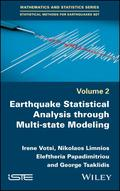John Wiley & Sons Earthquake Statistical Analysis through Multi-state ModelingEarthquake occurrence modeling is a rapidly developing research area. This book deals with its criti.. Product #: 978-1-78630-150-5 Regular price: \$104.67 \$104.67 Auf Lager

# Earthquake Statistical Analysis through Multi-state Modeling1. Auflage Januar 2019
180 Seiten, Hardcover
Wiley & Sons Ltd

ISBN: 978-1-78630-150-5
John Wiley & Sons

Jetzt kaufen

### Preis: 112,00 €

Preis inkl. MwSt, zzgl. Versand

Earthquake occurrence modeling is a rapidly developing research area. This book deals with its critical issues, ranging from theoretical advances to practical applications.

The introductory chapter outlines state-of-the-art earthquake modeling approaches based on stochastic models. Chapter 2 presents seismogenesis in association with the evolving stress field. Chapters 3 to 5 present earthquake occurrence modeling by means of hidden (semi-)Markov models and discuss associated characteristic measures and relative estimation aspects. Further comparisons, the most important results and our concluding remarks are provided in Chapters 6 and 7

List of Abbreviations ix

List of Symbols xi

Preface xv

Introduction xix

Chapter 1. Fundamentals on Stress Changes 1

1.1. Introduction 1

1.2. Stress interaction 4

1.3. Stress changes calculation 12

1.4. Modeling of Coulomb stress changes for different faulting types 15

1.4.1.DeltaCS for strike-slip faulting 15

1.4.2.DeltaCS for dip-slip faulting 16

1.5. Seismicity triggered by stress transfer 21

1.5.1. Triggering of strong earthquakes 21

1.5.2. Aftershock triggering 23

1.5.3. Triggering of mining seismicity 28

1.6. Discussion on stress interaction 31

Chapter 2. Hidden Markov Models 35

2.1. Introduction 35

2.2. Hidden Markov framework 37

2.3. Seismotectonic regime and seismicity data 42

2.4. Application to earthquake occurrences 44

2.4.1. Two hidden states and three observation types 45

2.4.2. Three hidden states and three observation types 48

2.4.3. Model selection and simulation 50

2.4.4. Steps number for the first earthquake occurrence 53

2.5. Conclusion 54

Chapter 3. Hidden Markov Renewal Models 57

3.1. Introduction 57

3.2. Semi-Markov framework 58

3.3. Hidden Markov renewal framework 65

3.4. Modeling earthquakes in Greece 66

3.4.1. Hitting times and earthquake occurrence numbers 69

3.5. Conclusion 73

Chapter 4. Hitting Time Intensity 75

4.1. Introduction 75

4.2. DTIHT for semi-Markov chains 76

4.2.1. Statistical estimation of the DTIHT 78

4.3. DTIHT for hidden Markov renewal chains 83

4.3.1. Statistical estimation of the DTIHT 85

4.4. Conclusion 87

Chapter 5. Models Comparison 89

5.1. Introduction 89

5.2. Markov framework 90

5.2.1. HMM case 92

5.2.2. HMRM case 92

5.3. Markov renewal framework 93

5.3.1. HMM case 95

5.3.2. HMRM case 96

5.4. Conclusion 97

Discussion & Concluding Remarks 99

Appendices 105

Appendix 1 107

Appendix 2 113

Appendix 3 117

References 119

Index 137
Irene Votsi is Assistant Professor of Statistics at the Manceau Laboratory of Mathematics, Le Mans University, France.

Nikolaos Limnios is Professor of Applied Mathematics at the University of Technology of Compiègne, Sorbonne University, France.

Eleftheria Papadimitriou is Professor of Seismology at the Aristotle University of Thessaloniki, Greece.

George Tsaklidis is Professor of Probability and Statistics at the Aristotle University of Thessaloniki, Greece.

N. Limnios, University of Technology of Compiegne, France